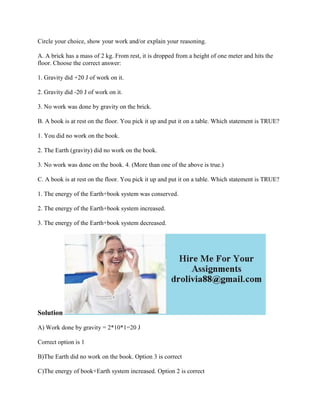Successfully reported this slideshow.×

## Check these out next

A. A brick has a mass of 2 kg. From rest, it is dropped from a height of one meter and hits the floor. Choose the correct answer:
1. Gravity did +20 J of work on it.
2. Gravity did -20 J of work on it.
3. No work was done by gravity on the brick.
B. A book is at rest on the floor. You pick it up and put it on a table. Which statement is TRUE?
1. You did no work on the book.
2. The Earth (gravity) did no work on the book.
3. No work was done on the book. 4. (More than one of the above is true.)
C. A book is at rest on the floor. You pick it up and put it on a table. Which statement is TRUE?
1. The energy of the Earth+book system was conserved.
2. The energy of the Earth+book system increased.
3. The energy of the Earth+book system decreased.
Solution
A) Work done by gravity = 2*10*1=20 J
Correct option is 1
B)The Earth did no work on the book. Option 3 is correct
C)The energy of book+Earth system increased. Option 2 is correct
.

A. A brick has a mass of 2 kg. From rest, it is dropped from a height of one meter and hits the floor. Choose the correct answer:
1. Gravity did +20 J of work on it.
2. Gravity did -20 J of work on it.
3. No work was done by gravity on the brick.
B. A book is at rest on the floor. You pick it up and put it on a table. Which statement is TRUE?
1. You did no work on the book.
2. The Earth (gravity) did no work on the book.
3. No work was done on the book. 4. (More than one of the above is true.)
C. A book is at rest on the floor. You pick it up and put it on a table. Which statement is TRUE?
1. The energy of the Earth+book system was conserved.
2. The energy of the Earth+book system increased.
3. The energy of the Earth+book system decreased.
Solution
A) Work done by gravity = 2*10*1=20 J
Correct option is 1
B)The Earth did no work on the book. Option 3 is correct
C)The energy of book+Earth system increased. Option 2 is correct
.